#### You may also like### Euler's Squares

Euler found four whole numbers such that the sum of any two of the numbers is a perfect square...### Odd Differences

The diagram illustrates the formula: 1 + 3 + 5 + ... + (2n - 1) = n² Use the diagram to show that any odd number is the difference of two squares.### Tree Tops

Can you make sense of information about trees in order to maximise the profits of a forestry company?

# Under the Ribbon

##### Age 14 to 16 Challenge Level:

Many people thought that the middle position would be best - that the cube size which only just fits under in the middle would not fit under anywhere else . . . . however no one has yet offered a reason for believing this . .A spreadsheet may help, though only if we can reason correctly about what the spreadsheet shows. You may like to look at a spreadsheet created for this problem.

Excel file : UnderRibbon

First you need to select a size of cube, the final column will then show the amount of ribbon needed for that cube placed at various positions along the metre length. However you will still need to reason quite carefully before you can be sure that the centre is the best position.Once you are happy about that, the Excel file has a second sheet called 'Cube in the Middle' (see the blue tab at the bottom of the page).This sheet represents a 'trial and improvement' method for a cube placed at the mid-point. You select the cube size to start at and also the size of the increase row on row, the final column will report the total length of ribbon required. That needs to be as close to 104 as you can manage without exceeding it. Although no one really accounted for their choice of the middle position, some good work was done with that position assumed.

Logan from McQueen High School, Colin from Northcote College, Keith from Nelson and Colne, and Sudheendra from Bangalore, all successfully arrived at a cube side length of 13.3178 by solving equations.

Here's how their algebra went :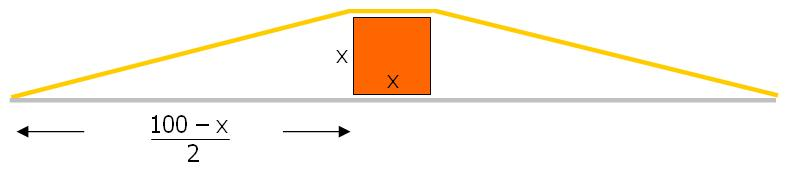x is used to represent the side length for the cube, and the space either side of the cube is expressed in terms of x.

The slope length of the ribbon can then be expressed in terms of x, by using Pythagoras, and hence the whole ribbon length for a cube of side x is :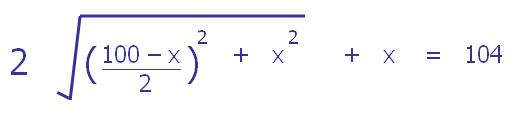This expression has been set equal to 104 so that we can try to solve the equation for x

Removing x from each side :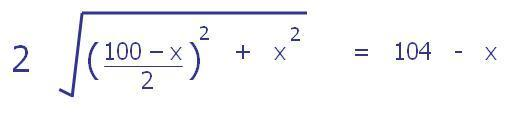Then squaring :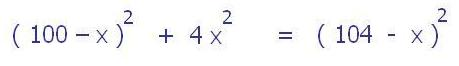Removing the brackets and tidying up :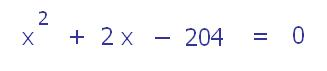And the formula for solving quadratic equations, which amounts to 'completing the square', then gives us a solution to the practical context : the cube side should be 13.3178 cm (4dp)

A negative solution might fit the equation but the expression is only being used to represent a context where x has a positive value.